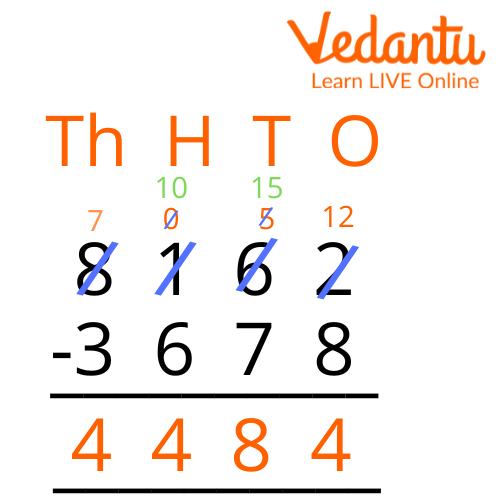Courses
Courses for Kids
Free study material
Free LIVE classes
More

# 4 Digit SubtractionLIVE
Join Vedantu’s FREE Mastercalss

## What is Subtraction?

Subtraction is an operation that is used to calculate the difference between two numbers. When a group of things is reduced in size by removing a few of them, the group becomes smaller. For example, suppose you bought 9 cupcakes for your birthday party and your guests ate 7 of them. You are now down to two cupcakes. This can be expressed as a subtraction expression: 9 - 7 = 2 and read as "nine minus seven equals two." We get 2 when we subtract 7 from 9 (9 - 7). In this case, we subtracted two numbers, 9 and 7, to get the difference of 2.

## What is 4-Digit Subtraction?

The difference between two 4-digit numbers is represented by 4-digit subtraction. Any number less than four digits can be removed from it as well. We know that the greater number should go higher and the smaller one should go down. The greater number at the top is known as the minuend, while the smaller number written below is known as the subtrahend. The difference between two numbers is the result of their subtraction. Both integers are inserted in their corresponding place value columns, one below the other, and then subtracted.

## Risks for 4-Digit Subtraction

For a 4-digit subtraction, we utilize the means given underneath.

Step 1: Place the numerals one on top of the other, with the minuend on top and the subtrahend directly below it.

Step 2: Arrange the numbers according to their place values of ones, tens, hundreds, and thousands.

Step 3: Begin subtracting digits from the ones column, then the tens column, hundreds column, then thousands column.

Step 4: Once the difference has been calculated, the corresponding solution is put beneath it. The difference between the two numbers is given by this.

## 4-Digit Subtraction Without Regrouping

4-digit subtraction without regrouping means the numbers are subtracted without any borrowing. In such cases, all the digits in the minuend are bigger than the digits in the subtrahend. Let us understand this using an example.

Example: Subtract 2719 from 3989.

Step 1: Arrange the numbers according to their place value. Start subtracting the digits in ones column. Now, 9 - 9 =0.

Step 2: Now , 8 - 1 = 7.

Step 3: So, 9 - 7 = 2.

Step 4: Now, let us subtract the digits in the thousands place. So, 3 - 2 = 1.4-Digit Subtraction Without Regrouping

## 4-Digit Subtraction with Regrouping

4-digit subtraction with regrouping is also known as borrowing subtraction. We regroup the integers and then subtract when any digit in the minuend (top row) is less than the corresponding digit in the subtrahend (bottom row). We borrow 1 number from the preceding column while regrouping to make that particular digit in the minuend larger than the corresponding digit in the subtrahend. Let me illustrate this with an example.

Step 1: Arrange the numbers as per their place esteem. Begin taking away the digits in one section. We can see that 2 is more modest than 8. In this way, we will get 1 from the tens segment which will make it 12. Presently, 12 - 8 = 4.

Step 2: After giving 1 to the one's segment in the next step, 6 becomes 5. Presently, let us deduct the digits during the tens place (5 - 7). Here, 5 is more modest than 7. Thus, we will acquire 1 from the hundreds segment. This will make it 15. Thus, 15 - 7 = 8.

Step 3: In sync 2 we had given 1 to the tens section, so we have 0 out of hundreds place. Here, 0 is more modest than 6, so we will acquire 1 from the large numbers section. This will make it 10. Thus, 10 - 6 = 4.

Step 4: Now, let us take away the digits in the place of the large number. After giving 1 to the hundreds section, 8 becomes 7. Thus, 7 - 3 = 4.

4-digit subtraction with pulling togetherSubtraction

Subsequently, the difference between the two given numbers is 8162 - 3678 = 4484.

## Summary

A 4-digit subtraction is the difference between a 4-digit number and another 4-digit number. Any number less than a four-digit number can also be subtracted from it. We know that the larger number should be placed on top and the smaller number should be placed below it. The larger number at the top is called the minuend and the smaller number written below is the subtrahend. The final result of subtracting two numbers is called their difference. Both numbers are placed below each other in their respective place value columns and then subtracted.

Last updated date: 21st Sep 2023
Total views: 110.1k
Views today: 2.10k

## FAQs on 4 Digit Subtraction

1. Is a 4-digit subtraction worksheet beneficial?

A 4-digit subtraction with regrouping worksheets is beneficial for improving a child's subtraction skills. Subtraction is an essential arithmetic skill that requires a clear understanding and conceptual knowledge.

2. What is the difference between subtraction and difference?

A "difference" is the result of a "subtraction". i.e. subtraction is an operation, and a difference is an element of a group.

3. Where do we use subtraction in our daily lives?

Subtraction is used in our day-to-day life. For example, if we want to know how much money we spent on the items that we bought, or, how much money is left with us, or, if we want to calculate the time left in finishing a task, we use subtraction.